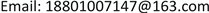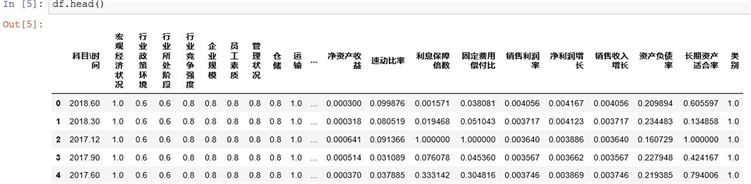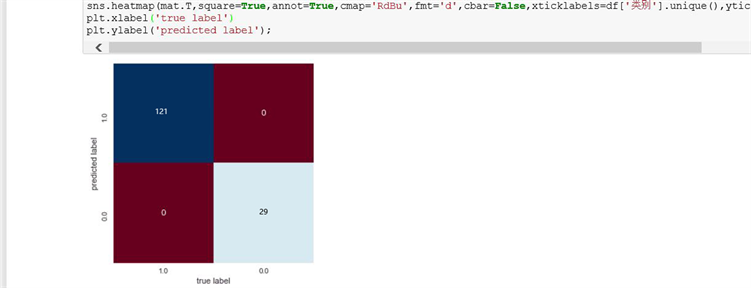﻿ 物流金融风险评估模型研究 Logistics Financial Risk Assessment Model Research

Management Science and Engineering
Vol. 08  No. 01 ( 2019 ), Article ID: 29180 , 8 pages
10.12677/MSE.2019.81009

Logistics Financial Risk Assessment Model Research

Mingming Fan, Shimin Wang

School of Computer & Information Engineering, Beijing Technology & Business University, BeijingReceived: Feb. 15th, 2019; accepted: Mar. 1st, 2019; published: Mar. 8th, 2019ABSTRACT

In this paper, the development of the core enterprise in the logistics financial system has strong guarantee for the stability of the supply chain, and the problem of financing of logistics enterprises has not been well solved, in which the difficult point of pain is not a large measure of the business ability of the bank, but the small sample problem is also a key factor in the failure of bank to analyze accurate analysis. This paper studies the risk assessment model of the core enterprise, namely the logistics enterprise loan, from the perspective of the bank in the supply chain finance. Oriented by the bank risk and the development of the logistics enterprise, this paper constructs a multi-index assessment model from the perspective of economics. Under the background of considering the enterprise size and operation status, this model uses the principal component analysis to determine the stability of the supply chain and upstream and downstream enterprises and other factors to confirm the evaluation index of the logistics enterprise. Finally through the concrete example simulation test, the results show that the bank under the restricted condition of the small sample can better comprehensive evaluation of logistics enterprises to financing risk.

Keywords:Logistics Enterprises, Internal Supply Chain, Principal Component Analysis, Support Vector Machine1. 引言

2. 物流金融信用风险指标体系构建Table 1. Logistics financial risk assessment index systemTable 2. Three-level indicators and index descriptionTable 3. Indicators with large correlation coefficient

3. 基于支持向量机物流金融信用风险评估模型

3.1. 数学建模

1) 线性分类：

$\mathrm{max}Q\left(\alpha \right)={\sum }_{i=1}^{n}{\alpha }_{i}-\frac{1}{2}{\sum }_{i=1}^{n}{\sum }_{j=1}^{n}{\alpha }_{i}{a}_{j}{y}_{i}{y}_{j}{x}_{i}^{\text{T}}{x}_{j}$

s.t. ${\sum }_{i=1}^{n}{a}_{i}{y}_{i}=0$ ，且 ${a}_{i}\ge 0,i=1,2,\cdots ,n$

$\mathrm{max}Q\left(\alpha \right)=\frac{1}{2}{\sum }_{i=1}^{n}{\sum }_{j=1}^{n}{\alpha }_{i}{a}_{j}{y}_{i}{y}_{j}{x}_{i}^{\text{T}}{x}_{j}-{\sum }_{i=1}^{n}{\alpha }_{i}$

s.t. ${\sum }_{i=1}^{n}{a}_{i}{y}_{i}=0$ ，且 ${a}_{i}\ge 0,i=1,2,\cdots ,n$

s.t. ${\sum }_{i=1}^{n}{a}_{i}{y}_{i}=0$ ，且 $0\le {a}_{i}\le C,i=1,2,\cdots ,n$

$\mathrm{min}\omega ,b,\xi \varphi \left(\omega ,x\right)=\frac{1}{2}{\omega }^{\text{T}}\omega +C{\sum }_{i=1}^{n}{\xi }_{i}$ s.t. 

2) 非线性分类：

$\mathrm{max}Q\left(\alpha \right)={\sum }_{i=1}^{n}{\alpha }_{i}-\frac{1}{2}{\sum }_{i=1}^{n}{\sum }_{j=1}^{n}{\alpha }_{i}{a}_{j}{y}_{i}{y}_{j}K\left({x}_{i},{x}_{j}\right)$

s.t. ${\sum }_{i=1}^{n}{a}_{i}{y}_{i}=0$ ，且 $0\le {a}_{i}\le C,i=1,2,\cdots ,n$

$f\left(x\right)=\mathrm{sgn}\left({\sum }_{i=1}^{n}{a}_{i}^{*}{y}_{i}K\left({x}_{i},{x}_{j}\right)+{b}^{*}\right)$

3.2. 模型构建

1) 数据归一化：

${x}_{i}=\frac{{x}_{i}-{\mathrm{min}}_{i}}{{\mathrm{max}}_{i}-{\mathrm{min}}_{i}}$

2) 核函数的选取和参数的选择：

4. 实证研究Figure 1. Support vector machine output resultsFigure 2. Test set accuracy and accuracy

5. 总结

Logistics Financial Risk Assessment Model Research[J]. 管理科学与工程, 2019, 08(01): 64-71. https://doi.org/10.12677/MSE.2019.81009

1. 1. 孔凡超, 窦志武, 熊琪. 第三方物流视角下物流金融风险评估研究[J]. 物流工程与管理, 2013, 35(3): 60-62.

2. 2. 赵云. 商业银行物流金融业务信用风险管理研究[D]: [硕士学位论文]. 天津: 天津理工大学, 2010.

3. 3. 汪涛. 经济新常态下中小企业互联网融资风险预警机制体系研究[J]. 经济师, 2018(4): 28-29, 32.